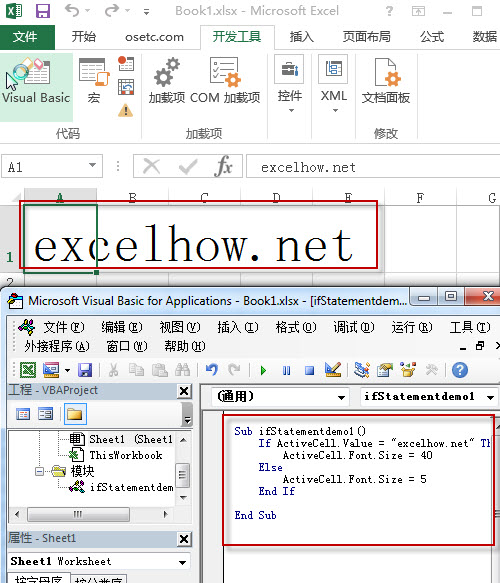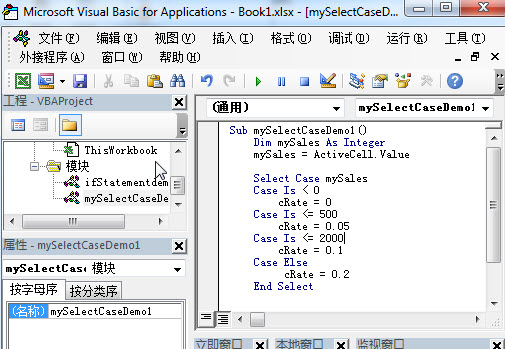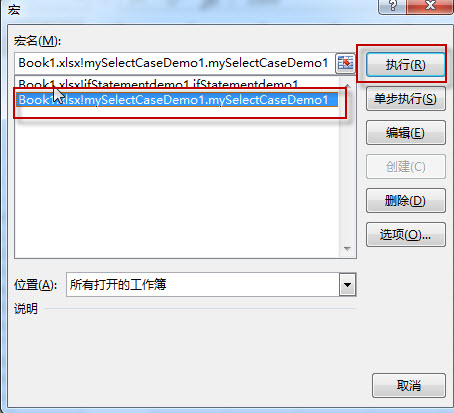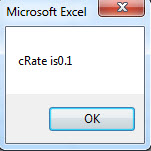# Excel VBA Decisions

This post will guide you how to use conditional statements in MS excel VBA. There are two most useful conditional statements as bellows: If …Then…Else and Select Case.

### IF…Then…Else Statement

This statement will test a condition and if the condition is true, then the conditional code will be executed. If the condition is false, then a different conditional code is executed.

The syntax of the IF …Then…Else statement is:

If conditional1 Then

Conditinal1 code (code will be executed if conditinal1 is true)

ElseIf Conditional2 Then

Conditinal2 code (code will be executed if conditinal2 is true)

Else

Code (if all of the above conditionals are false, this code will be executed)

End If

Example1: if the value of cell is “excelhow.net” and then set the font size to 40 or set the font size to 5.

Type the below VBA codes in the Visual Basic Editor:

```Sub ifStatementdemo1()
If ActiveCell.Value = "excelhow.net" Then
ActiveCell.Font.Size = 40
Else
ActiveCell.Font.Size = 5
End If
End Sub```### VBA Select Case Statement

The “Select Case” statement is similar to the If … Then … Else statement… it will check expression/variable if matches the case statement values, if yes, the code will be executed under this case statement.

The syntax of the Select Case statement is:

Select Case Expression

Case Value1

Case statement code1 (to be executed if expression equal to Value1)

Case Value2

Case statement code2 (to be executed if expression equal to Value2)

Case Else

Case statement else(to be executed if none of values match expression)

End Select

Example1:  VBA Select Case statement example

1# open visual Basic Editor, then insert a module and name as :mySelectCaseDemo1

2# enter into the below VBA codes in code window.

```Sub mySelectCaseDemo1()
Dim mySales As Integer
mySales = ActiveCell.Value
Select Case mySales
Case Is < 0
cRate = 0
Case Is <= 500
cRate = 0.05
Case Is <= 2000
cRate = 0.1
Case Else
cRate = 0.2
End Select
MsgBox "cRate is" & cRate
End Sub```3# back to workbook and run the above macro.